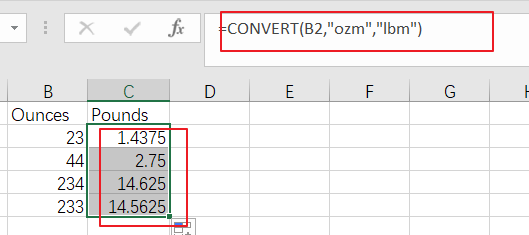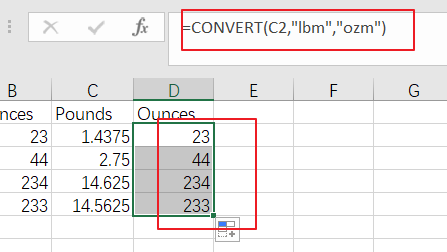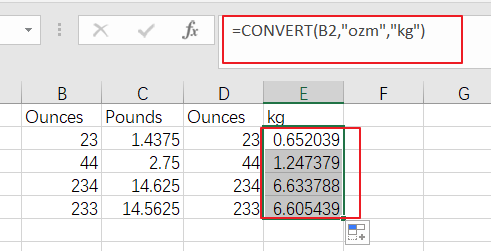# How to Convert Ounces to Pounds or Grams in Excel

This post will guide you how to convert ounces to pounds or grams with formula in Excel. How do I convert pounds to ounces or grams in Excel 2013/2016.

## Convert Ounces to Pounds

Assuming that you have a list of data in range B1:B4, in which contain values with Ounces data, and you wish to convert these Ounces to pounds. How to do it. You can use an formula based on the CONVERT function to convert it quickly. Like this:

`=CONVERT(B2,"ozm","lbm")`

You need to type this formula into a blank cell such as: Cell C2, and press Enter key on your keyboard, and then drag the AutoFill handle down to other cells to apply this formula.## Convert Pounds to Ounces

If you want to convert your range of cells with Pounds data to Ounces, you can use the below formula based on the CONVERT function:

`=CONVERT(C2,"lbm","ozm")`

You need to type this formula into a blank cell such as: Cell D2, and press Enter key on your keyboard, and then drag the AutoFill handle down to other cells to apply this formula.## Convert Ounces to kg

If you want to convert kg into Ounces for you range B2:B5, just use the following formula:

`=CONVERT(B2,"ozm","kg")`

You need to type this formula into a blank cell such as: Cell E2, and press Enter key on your keyboard, and then drag the AutoFill handle down to other cells to apply this formula.